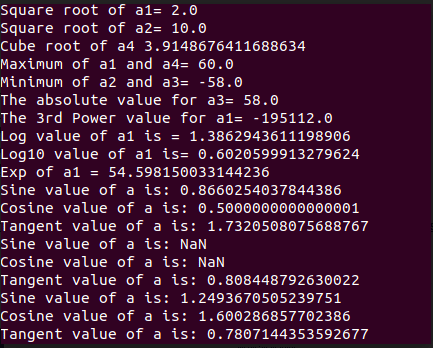# Math class in Java Example | Java Math Class Tutorial

Using the Java Math class, we can implement methods like min(), max(), avg(), sin(), cos(), tan(), round(), ceil(), floor(), abs(), pow(), floor(), round() etc.

## Math class in Java

Several times we need to perform some mathematical functions in our program. Sometimes we can create separate methods for the same, but while making large and complex problems, we do not have time for doing so. So, to implement these functions in our program, we can use the methods already defined in the Math class (which is present inside the java.lang.Math package).

## Importing Math Class

If we want to import Math class in java, we have to write the following code.

```java.lang.math
```

## Various Methods in Math Class

The following are the methods that you can directly implement in your program code using the Math class.

 Method Working Description Math.max() It takes two values as inputs and returns the maximum of two values. Math.min() It takes two values as inputs and returns the minimum of two values. Math.abs() It will take one value as input and return the absolute value for it. Math.round() It is used to round-of the decimal numbers to the nearest integer value. Math.sqrt() It takes one argument as input and returns the square root of that number. Math.cbrt() It takes one argument as input and returns the cube root of that number. Math.atan() It is used to return a trigonometric Arc Tangent value of the given value Math.hypot() This method takes two double values as the arguments and returns sqrt(x2+y2) without any intermediate overflow or underflow. Math.ceil() It is used in case of decimal or fraction values to find the smallest integer value that is greater than or equal to an argument passed to it. Math.floor() It is also used in case of decimal or fraction values but to find the largest integer value that is lesser than or equal to the argument passed to it. Math.getExponent() It is used to return an unbiased exponent used in the representation of a value. Math.log() It takes a double value as input and returns the natural logarithm of that double value. Math.log10() It takes a double value as input and is used to return the base 10 logarithm of that double value. Math.log1p() It takes a double value as input and returns the natural logarithm of that double value. Math.exp() It returns ‘e’ raised to the power of a double value, which is also known as the exponential of the number, where ‘e’ is Euler’s number, and it is approximately equal to 2.71828. Math.expm1() It returns ‘e’ raised to the power of a double value, which is also known as the exponential of the number subtracted one from it. Math.sin() It takes one double value as its argument and returns the trigonometric Sine value of a Given double value. Math.cos() It takes one double value as its argument and returns the trigonometric Cosine value of a Given double value. Math.tan() It takes one double value as its argument and returns a trigonometric Tangent value of the given double value. Math.asin() It is used to return a trigonometric Arc Sine value of the given value. Math.acos() It is used to return a trigonometric Arc Cosine value of the given value. Math.sinh() It takes one double value as its argument and returns the hyperbolic Sine value of a Given double value. Math.cosh() It takes one double value as its argument and returns the hyperbolic Cosine value of a Given double value. Math.tanh() It takes one double value as its argument and returns the hyperbolic Tangent value of a Given double value.

The following program code is an example that implements some of the methods of the Math class in Java:

```import java.lang.Math;

public class MathDemo {
public static void main(String[] args) {

double a1= 4;
double a2= 100;
double a3= -58;
double a4= 60;

System.out.println("Square root of a1= "+ Math.sqrt(a1));
System.out.println("Square root of a2= "+ Math.sqrt(a2));

System.out.println("Cube root of a4 "+ Math.cbrt(a4));

System.out.println("Maximum of a1 and a4= "+ Math.max(a1,a4));
System.out.println("Minimum of a2 and a3= "+ Math.min(a2,a3));

System.out.println("The absolute value for a3= "+ Math.abs(a3));

System.out.println("The 3rd Power value for a1= "+ Math.pow(a3,3));
System.out.println("Log value of a1 is = "+ Math.log(a1));
System.out.println("Log10 value of a1 is= "+ Math.log10(a1));
System.out.println("Exp of a1 = "+ Math.exp(a1)); //return a power of 2

// Now we will calculate trigonomatry values
//First we have convert one value to radian
// so here we will convert a4 to radian

System.out.println("Sine value of a is: " +Math.sin(a));

System.out.println("Cosine value of a is: " +Math.cos(a));

System.out.println("Tangent value of a is: " +Math.tan(a));

// return the trigonometric arc sine of a
System.out.println("Sine value of a is: " +Math.asin(a));

// return the trigonometric arc cosine value of a
System.out.println("Cosine value of a is: " +Math.acos(a));

// return the trigonometric arc tangent value of a
System.out.println("Tangent value of a is: " +Math.atan(a));

// return the hyperbolic sine of a
System.out.println("Sine value of a is: " +Math.sinh(a));

// return the hyperbolic cosine value of a
System.out.println("Cosine value of a is: " +Math.cosh(a));

// return the hyperbolic tangent value of a
System.out.println("Tangent value of a is: " +Math.tanh(a));
}
}```

See the following output.Finally, Math class in Java Example Tutorial is over.

## Recommended Posts

Java Dictionary Class Example

Nested class In Java Example

Java Type Casting Example

Java Binary Search Program

equalsIgnoreCase() In Java Example

This site uses Akismet to reduce spam. Learn how your comment data is processed.